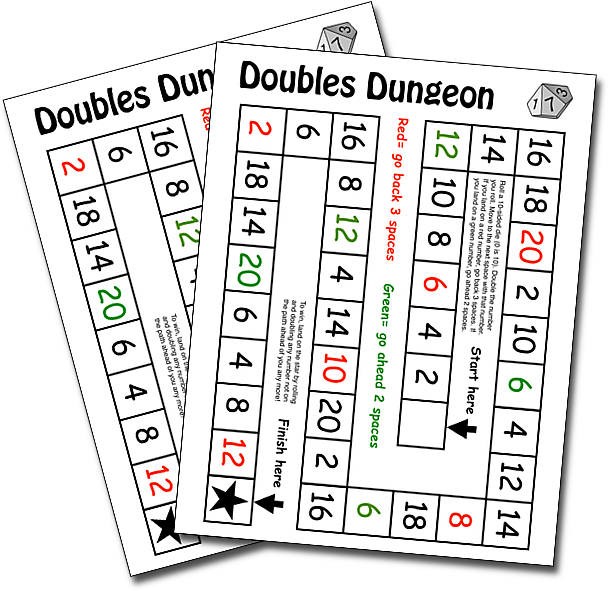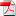# Doubles Practice Game

Doubles Dungeon. Free Printable Math Game. Arithmetical Board Game. Game to practice addition doubles facts. Free Mathematical Game. Mental arithmetic.Use the password worksheets.site to open the PDF file.

## 1. Doubles

Adding doubles, such as 4+4 or 9+9, is one important strategy for learning addition facts. Pairs of numbers formed with the same number (8 + 8) are generally easier to remember than other addition pairs. For this reason they are can be used as scaffolding for figuring out other results. Doubles don't usually require special instruction but some games such as dominoes or frequent situations of daily life can be used as examples in case of difficulty:

• The eyes, 1 + 1.
• The wheels of a car. The legs of a table, 2 + 2.
• Two tricycles. Two trimesters. Two triangles, 3 + 3.
• The rear wheels of a truck. Two dogs, 4 + 4.
• The fingers of the hands, 5 + 5.
• Two half dozen eggs, 6 + 6
• The days of two weeks, 7 + 7.
• Two spiders. 8 + 8.
• Two tic-tac-toe boards. 9 + 9.
• Ten fingers and ten toes. 10 + 10.

Children come to master doubling with such certainty an accuracy that this tool becomes a more general calculation strategy, Doubling is practically an operation in itself. Many people resort to doubling every time they have to multiply by two.

## 2. Doubles Plus One

It is a way of taking doubling to the next level. For example 5 + 6. To solve them, it is enough to increase in one unit the value of the double (5 + 6 = 5 + 5 + 1). Help kids recognize when addition facts are "next door neighbors", just one number apart from one another. The memory rule here should be, "When numbers are neighbors, get doubles to help".

## 3. Missing number

When you are facing a pair of almost neighboring numbers, numbers between which there is another hidden number, 7 + 9 or 6 + 8, then, it is possible to solve the situation by finding the double of the missing number, 8 in 7 + 9 or 7 in 6 + 8. 7 + 9 becomes 8 + 8, and 6 + 8 becomes 7 + 7.

## 4. Doubles Practice Game

This print and play game comes in a PDF file. It contains a Board game sheet with a dungeon full of doubles ready to be crawled. It's a variation of the popular Snake & Ladders game.

Roll a 10-sided die (0 is 10). Double the number you roll. Move to the next space with that number. If you land on a red number, go back 3 spaces. If you land on a green number, go ahead 2 spaces.

To win, land on the star by rolling and doubling any number not on the path ahead of you any more!